# Break Chart Y Axis

This post will guide you how to break the Chart Y axis in an Excel worksheet. How do I make a chart with a break Y axis in Excel.

## Break Chart Y Axis

For example, Assuming that you have the data in a range (B2:B5) from 10-50 and another range (C2:C5) from 60-90. You have create a line chart based on the data in your worksheet. And now you want to break the Y-axis in the existing chart. How to achieve it. Just do the following steps:

#1 select the range of cells A1:C5 as the source data of the chart.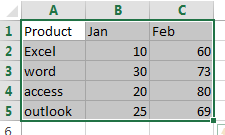#2 go to INSERT tab, click 2-D Line button under Charts group to create a Line chart.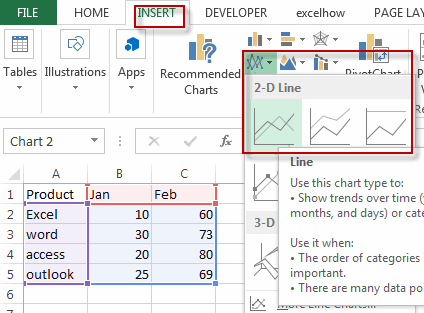#3 right click Data Series at the bottom of the chart, select the Format Data Series from the drop-down menu list.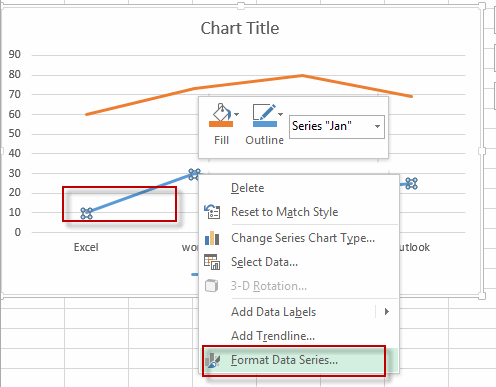#4 select the Secondary Axis radio button in the SERIES OPTIONS section under Format Data Series pane.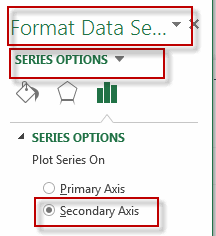#5 the current chart added one more Y-Axis and it also called the secondary vertical axis.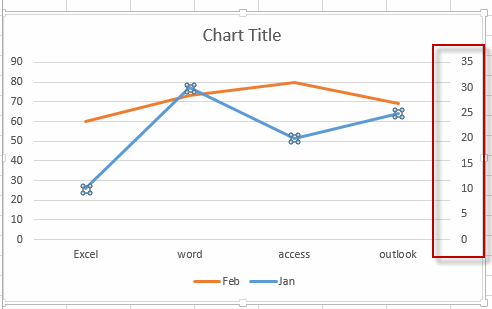#6 right click on the secondary Y axis and select the Format Axis from the drop down list.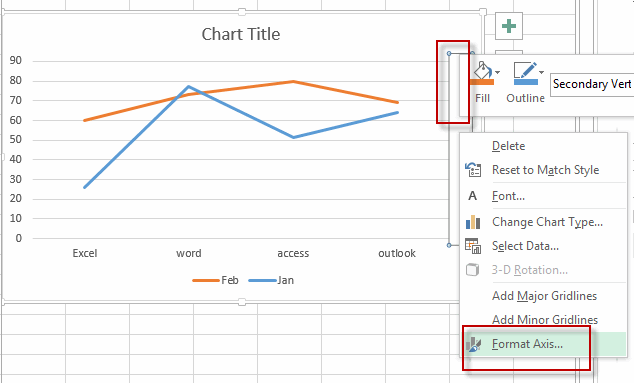#7 under the Format Axis pane, type the number 50 into the Maximum box, and type the [<=35] 0;;; into the Format Code text box in the Number section, and click Add button.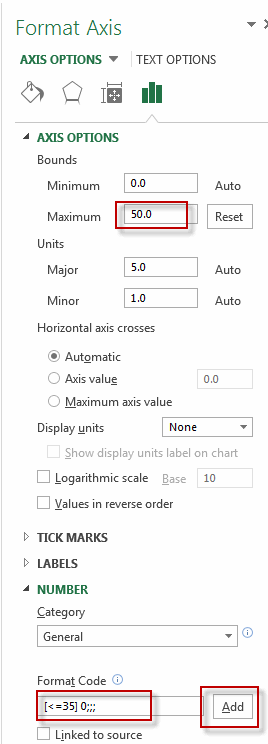#8 right click on the primary Y axis in the chart, and select the Format Axis from the drop down list. Type the [>=60]0;;; into the Format Code text box in the Number section, and click Add button.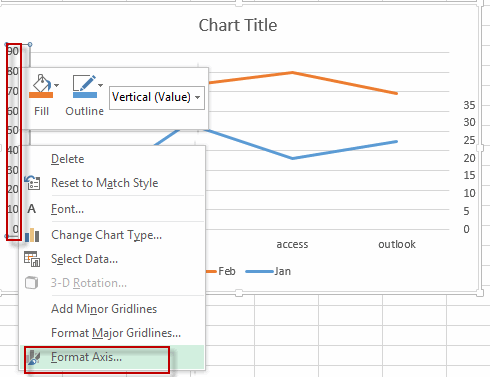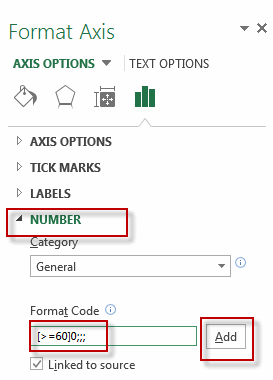#9 you will see that the chart Y axis has been broken in your current chart.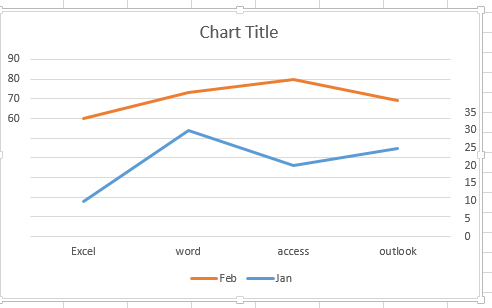Related Posts
If Cell is This Value or That Value

IF function is frequently used in Excel worksheet to return you expect “true value” or “false value” based on the result of logical test. If you want to see if a cell is A or B, and if one of ...

If Value is Greater Than A Certain Value

IF function is frequently used in Excel worksheet to return you expect “true value” or “false value” based on the logical test result. If you want to see if a value in one cell is greater than a specific value, ...

If Cell is Not Blank

IF function is frequently used in Excel worksheet to return you expect “true value” or “false value” based on the result of created logical test. If you want to see if a cell is blank or not, and leave some ...

VBA Macro For VLOOKUP From Another Sheet

In the previous post, you should know that how to fix or remove the #N/A error when using VLOOKUP formula to lookup value from another sheet. And this post will show you how to use VBA code to vlookup data ...

If Cell is Blank

IF function is frequently used in Excel worksheet to return you expect “true value” or “false value” based on the result of created logical test. If you want to see if a cell is blank or not, and leave some ...

If Cell Equals Certain Text String

IF function is frequently used in Excel worksheet to return you expect “true value” or “false value” based on the result of created logical test. If you want to see if cell equals a certain text string like “Win”, you ...

If Cell Contains Either Text1 or Text2

IF function is frequently used in Excel worksheet to return “true value” or “false value” based on the logical test result. If you want to see if cell contains certain substring1 like “abc” or substring2 like “def”, and returns true ...

If Cell Contains Certain Text OR Equals Certain Text

IF cell equals certain text IF function is frequently used in Excel worksheet to return “true value” or “false value” based on the logical test result. If you want to test values to see if they equal certain text like ...

VLOOKUP From Another Sheet Not Working

In the previous post, you should know that how to fix or remove the #N/A error when using VLOOKUP formula to lookup value from another sheet. And this post will show you reasons why your VLOOKUP formula is not working ...

If Cell Begins with One of Three Supplied Characters

If you want to test values to see if they begin with some given specific characters like “x”, ”y”, or “z”, you can create a formula with COUNTIF and SUM functions to return results. EXAMPLE You can see “TRUE” or ...

Sidebar